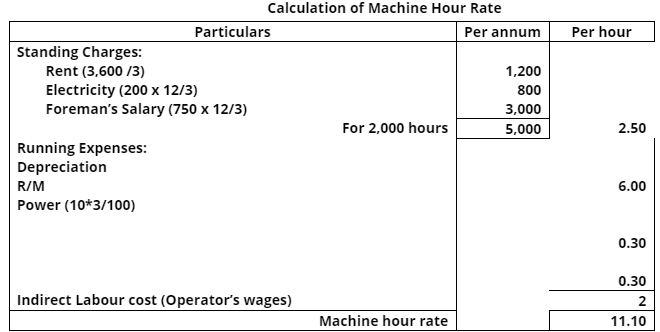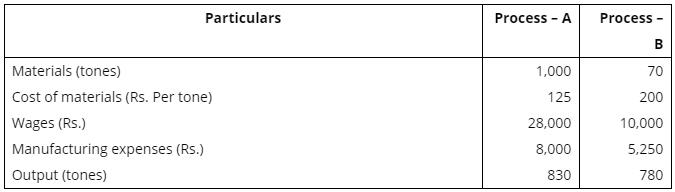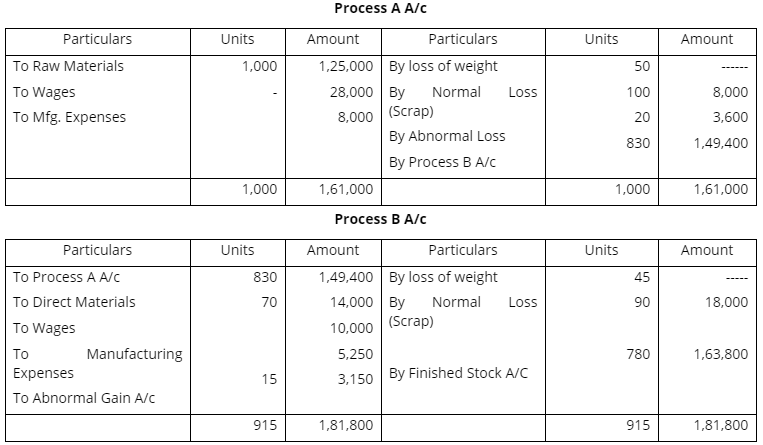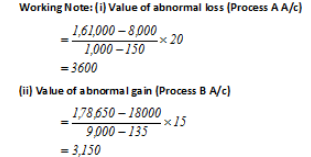Courses

# Cost Accounting Solved Question Papers (Part - 2) B Com Notes | EduRev

## B Com : Cost Accounting Solved Question Papers (Part - 2) B Com Notes | EduRev

The document Cost Accounting Solved Question Papers (Part - 2) B Com Notes | EduRev is a part of the B Com Course Cost Accounting.
All you need of B Com at this link: B Com

Ques 4. (a) The following are the information in respect to a worker who has manufactured 240 articles during the last week of December 2017:
Working hours during the week are 48 hours, standard rate Rs. 5 per hour and standard time to manufacture an article is 15 minutes.
Calculate his gross wages for the week according to:
(1) Piecework with guaranteed weekly wages;
Ans:
Time Take = 48 Hours
Time Allowed based on actual output = 15 mins * 240 = 3600 mins or 60 hours
Time Saved = 60 – 48 = 12 Hours
Piece produced per hour = 60/15 = 4 Units
Rate Per piece = 5/4 = Rs. 1.25
(1) Piece work with guaranteed weekly wages = Piece produced * Rate per piece
= 240*1.25
= Rs. 300
Or
Guaranteed weekly wages = 48*5 = 240
Whichever is higher is his actual wages i.e., Rs. 300
(2) Rowan Premium Plan = TT*Rate per Hour + TS/ST (TT*Rate per hour)
= 48*5+ 12/60(48*5) = Rs. 288
(3) Halsey Premium plan = TT*Rate per hour + 50% (TS*Rate per hour)
= 48*5+50% (12*5) = Rs. 270

(b) (1) Describe the essential characteristics of a good system of wage payment.
Ans: An ideal incentive plan must possess the following features:
(a) Simplicity - The plan should be simple to understand and operate. Who should be able to calculate their wages without any difficulty?
(b) Acceptability - It should be acceptable to workers as well as the employer.
(c) Flexibility - The incentive plan should be flexible to introduce nice changes.
(d) Quality - The plan should ensure the quality of the output. Workers should be discouraged to speed up the work to earn more wages at the cost of quality.
(e) Stability - The plan should give a stable earnings over a period of time, minimum but adequate wage must be ensured.
(f)  Wide coverage - It should cover the maximum number of workers. 1 direct as well as indirect worker should be covered.
(g) No restriction on earnings - The plan should not have any restriction earnings of workers. They should be allowed to earn as much as they can.
(h) Investigation and evaluation - The plan should be based on scientific investigation and evaluation to produce good result. Standard time should fix on the basis of time and motion study.
(i) Increasing output and lowering cost of production - It should aim increasing output and lowering cost of production.
(j) Motivating to earn more - The plan should motivate the workers increase their efficiency and earn more.
The success of an incentive plan depends on the mutual cooperation a understanding between employer and employees.

(2) Describe with illustration the salient features of Rowan Plan and Halsey Plan.
Ans: The Halsey premium plan: This system is known as fifty-fifty plan. It was introduced by F.A. Halsey, an American engineer. Under this method a standard time is fixed for the performance of each job; worker is paid for actual time taken at an hourly rate plus 50% of time saved as bonus. Total wages under this scheme is calculated with the help of the following formula:
Earnings = Time taken x Rate per hour + 50% (Time saved x Rate per hour)

Principles/Features of Halsey Premium Scheme: Under this plan,
a. Time rate is guaranteed and the worker gets the guaranteed irrespective of whether he completes the job within the time also takes more time to do it.
b. Standard time and standard work are fixed for the job or operation in advance;
c. The workers producing more than the standard, or the workers completing the work in less than the standard time fixed, get bonus in addition to the ordinary time wage.
d. The bonus or the premium, by whatever name called, is 30 to 70 percent of the wages of time saved, the usual percentage being 50%,
e. Workers who fail to reach the prescribed standard get the time wages.
f. Labour cost per unit of output decreases. The employer also shares the benefit of efficiency which induced him to improve the method and equipment.
Rowan System or Rowan Plan: The scheme was introduced in 1901 by David Rowan of Glasgow, England. The wages are calculated on the basis of hours worked where as the ‘bonus is that proportion of the wages of time taken which the time saved bears to the standard time allowed’. Total wages under this scheme is calculated with the help of the following formula:
Earnings = Time taken x Rate per hour + Time saved / Standard time (Time taken x Rate per hour)
The main principles/features of Rowan plan are:
a. Time rate is guaranteed and the worker gets the guaranteed irrespective of whether he completes the job within the time also takes more time to do it.
b. Standard time and standard work are fixed for the job or operation in advance;
c. The workers producing more than the standard, or the workers completing the work in less than the standard time fixed, get bonus in addition to the ordinary time wage.
d. Bonus is based on that proportion of the time wages which the time saved bears to the standard time.
e. Workers who fail to reach the prescribed standard get the time wages.
f. Labour cost per unit of output decreases. The employer also shares the benefit of efficiency which induced him to improve the method and equipment.
g. Wages per hour increases but in the same proportion as the output.

Ques 5: (a) From the following information, compute machine hour rate of a machine in a shop consisting of 3 machines occupying equal floor space. The estimated working hours per year are fixed at 2500 hours in which normal idle time is estimated at 20% of the standard time:

 Rent and taxes of the shop per annum – Rs. 3,600General Electricity for the shop per month – Rs. 200Repairs and maintenance expenses for the machine per annum – Rs. 600Rate of power charges for 100 units (the machine consuming 10 units per hour) – Rs. 3Foreman’s salary for supervising all the machines per month – Rs. 750Indirect labour cost – Rs. 2 per hour for the machine.The machine cost – Rs. 1,30,000.Scrap value is estimated at Rs. 10,000.

Estimated life is 10 years. The foreman devotes equal attention for each machine in the shop.
Ans:Normal working hours = 2,500 – 20% = 2,000
Or
(b) What factors would you consider for determining the overhead absorption rate? Explain the causes of over and under-absorption of overheads.
The most important step in the overhead accounting is ‘Absorption’ of overheads. CIMA defines absorption as, ‘the process of absorbing all overhead costs allocated or apportioned over a particular cost center or production department by the units produced.’ In simple words, absorption means charging equitable share of overhead expenses to the products. As the overhead expenses are indirect expenses, the absorption is to be made on some suitable basis. The basis is the ‘absorption rate’ which is calculated by dividing the overhead expenses by the base selected. A base selected may be any one of the basis given below. The formula used for deciding the rate is as follows,

The methods used for absorption are as follows:
(a) Direct Material Cost: Under this method, the overheads are absorbed on the basis of percentage of direct material cost. The following formula is used for working out the overhead absorption percentage: Budgeted or Actual Overhead Cost/ Direct Material Cost X 100
(b) Direct Labor Cost Method: This method is used in those organizations where labor is a dominant factor in the total cost. Under this method, the following formula is used for calculating the overhead absorption rate: Budgeted or Actual Overheads/ Direct Labor Cost X 100
(c) Prime Cost Method: This method is an improvement over the first two methods. Under this method, the Prime Cost is taken as the base for calculating the percentage of absorption of overheads by using the following formula: Budgeted or Actual Overheads/ Prime Cost X 100
(d) Production Unit Method: This method is used when all production units are similar to each other in all respects. Total overhead expenses are divided by total production units for computing the rate per unit of overheads and overheads are absorbed in the product units. If a firm produces more than one products and if they are not uniform to each other, equivalent units are calculated to find out the rate of overheads per unit. The formula of absorption of overheads is as follows: Overhead absorption rate = Budgeted or Actual Overheads/Production Units
(e) Direct Labor Hour Method: Under this method, the rate of absorption is calculated by dividing the overhead expenses by the direct labor hours. The formula is as follows. Budgeted or Actual Overhead Expenses/Direct Labor Hours
(f) Machine Hour Rate: Where machines are more dominant than labor, machine hour rate method is used. CIMA defines machine hour rate as ‘actual or predetermined rate of cost apportionment or overhead absorption, which is calculated by dividing the cost to be appropriated or absorbed by a number of hours for which a machine or machines are operated or expected to be operated’. In other words, machine hour rate is the cost of operating a machine on per hour basis. The formula for calculating the machine hour rate is, Budgeted or Actual Overhead Expenses/ Machine Hours
(g) Selling Price Method: In this method, selling price of the products is used as a basis for absorbing the overheads. The logic used is that if the selling price is high, the product should bear higher overhead cost. Ratio of selling price is worked out and the overheads are absorbed.
Over or under absorption of overheads meaning:
Overhead expenses are usually applied to production on the basis of predetermined rates. The pre-determined rate may present estimated or actual cost. The actual overhead cost incurred and overhead applied to the production will seldom be the same. But due to certain reasons the difference between two may arise.
Over absorptions: If the amount applied exceeds, the actual overhead, it is said to be an over absorption of overheads.
Under absorption: If the amount applied is short fall of the actual overhead in production it is said to be the under absorption of overheads. The over or under absorption of overheads may be termed as overhead variance.
Reason of over or under-absorption of overheads: The under or over-absorption of overhead arises due to following reasons:
b. Overhead may change due to change in method of production.
c. The seasonal fluctuation in overhead cost in some industries.
d. Under utilization of available capacity, unexpected change in the volume of out put.
e. Valuation of work in progress in wrong process.

Ques 6: (a) A product of a manufacturing concern posses through two processes A and B and then to finished stock. It is ascertained that in each process 5% of the total weight is lost and 10% is scrap, which from processes A and B realizes Rs. 80 per tone and Rs. 200 per tone respectively. The following are the figures relating to both the processes:Prepare the Process Cost Accounts showing cost per tones of each process. There was no work-in-progress in any process.
Ans:(b) Define job costing. Where is it applied?
Ans:
Job Costing: Job costing is designed to accumulate cost data for a manufacturing firm which produces goods to specific order. It is also known as specific orders costing or production order costing. Under this method of costing, each job, batch or contract is treated as a cost unit and costs are collected and built up accordingly.
According to “ICMA”, London, it is that category of basic costing method which is applicable where the work consists of separate contract job or batches each of which is authorized by specific order or contract. It is followed by manufacturing and non-manufacturing concerns.
It is employed in industries in which:
(a) A production is done on the basis of customer’s own specifications.
(b) Products are manufactured in distinguishable lots.
(c) Products are not uniform.
(d) It is practical to maintain a separate record of each lot from the time production is begun until it is completed.
Following is the list of concerns which generally employ job costing method.
(a) Printing Work.
(b) Design Engineering Concerns.
(c) Repair Works.
(d) Construction companies.
(e) Furniture makers.
(f) Hardware industry.
(g) Automobile garages.
(h) Interior decoration etc.

Offer running on EduRev: Apply code STAYHOME200 to get INR 200 off on our premium plan EduRev Infinity!

## Cost Accounting

131 videos|139 docs

,

,

,

,

,

,

,

,

,

,

,

,

,

,

,

,

,

,

,

,

,

;